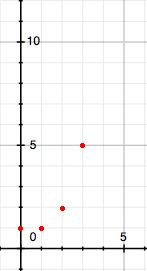SEARCH HOMEMath Central Quandaries & QueriesQuestion from Sang, a teacher: How to find the derivative of x! and integral of x!Hi Sang,

$y = f(x) = x!$ is not a differentiable function so it has no derivative. It also has no integral. The domain of $f(x) = x!$ is all non-negative integers. Its graph looks like$f(4) = 4! = 24, f(5) = 120$ and so on.

I hope this helps,
PennyMath Central is supported by the University of Regina and the Imperial Oil Foundation.